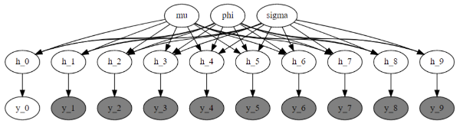# Render_model - reflection of the sequential dependency in HMM

Hello,

I am trying to use the “pyro.markov” in order to model a hidden Markov model. My model follows the exact structure as below code. Now, here is the problem that although the outputs key params make sense and distinguish between different steps of “x_t”, I wonder if the “pyro.markov” simulates the behavior that I expect from the HMM correctly or not. I doubt the proper functionality of this object, as the “render_model” that I receive does not reflect any connections (with arrows) between the latent variables of “x_t” (sequential that can be seen between the latents parameters in HMM). Am I missing something in the usage of “pyro.markov”? Or the connection holds at each step, but the render cannot reflect it? I would be grateful if you could help me with this confusion. Thank you in advance!

def state_space_model(data, N=1, T=2, prior_drift=0.):
# global rvs
drift = pyro.sample(‘drift’, dist.Normal(prior_drift, 1))
vol = pyro.sample(‘vol’, dist.LogNormal(0, 1))
uncert = pyro.sample(‘uncert’, dist.LogNormal(-5, 1))

``````latent = torch.empty((T, N))
with pyro.plate('data_plate', N) as n:
x0 = pyro.sample('x0', dist.Normal(drift, vol))  # or whatever your IC might be
latent[0, n] = x0

for t in pyro.markov(range(1, T)):
x_t = pyro.sample(f"x_{t}",dist.Normal(latent[t - 1, n] + drift, vol))
y_t = pyro.sample(f"y_{t}",dist.Normal(x_t, uncert),obs=data[t - 1, n])
latent[t, n] = x_t

return pyro.deterministic('latent', latent)
``````

pyro.render_model(model, model_args=(data))

What does your `render_model()` output look like?

Note `pyro.markov` is really only useful in discrete models using enumeration. In continuous Pyro models, Markov dependencies are automatically detected by automatic differentiation. So you could replace your loop with a simple `for t in range(1, T)`.

Note on the forum you can format code using triple back ticks
````python`
`insert code here`
`````

1 Like

Thank you so much for the quick response and recommendation. This is the render that I see:In my case, the pyro.markov loop is defined for the latent parameters of “h” and “y”, through the following code. I actually expected to see the arrows between the "h_{t}"s, but the rendering seems not to capture the relations as it is done through a transition variable “latent”.

I altered the code and just used a simple loop instead of the Markov loop. But, the same problem still exists and the render did not change.

If I understand correctly, the existence of ‘latent’ parameter (which is equal to the “h_{t-1}”) is why I cannot see the sequential arrows between "h_{t}“s, right? As I am new to the pyro, I dont know how to implement the HMM without this extra variable of “latent”, which makes the render not to follow the sequence which happening in the HMM. I tried the format of f"h_{t-1}” within the code instead of the latent[t-1,0], but it did not work.

``````for t in range(1, T):
h_t = pyro.sample(f"h_{t}", dist.Normal(mu+phi*(latent[t-1,0]-mu), sigma))
y_t = pyro.sample(f"y_{t}", dist.Normal(0.0, torch.exp(h_t/2)), obs=data[t])
latent[t, 0] = h_t
latent[t, 1] = y_t
return latent
``````

Oh I think the issue is that you’re using the `latent` for intermediate storage, and that’s breaking tracing. I’d recommend (i) avoiding reading from `latent` and even (ii) detaching before writing to it:

``````def state_space_model(data, N=1, T=2, prior_drift=0.):
# global rvs
drift = pyro.sample(‘drift’, dist.Normal(prior_drift, 1))
vol = pyro.sample(‘vol’, dist.LogNormal(0, 1))
uncert = pyro.sample(‘uncert’, dist.LogNormal(-5, 1))

latent = torch.empty((T, N))
with pyro.plate('data_plate', N) as n:
x_t = pyro.sample('x0', dist.Normal(drift, vol))  # or whatever your IC might be
latent[0, n] = x_t.detach()

for t in pyro.markov(range(1, T)):
x_t = pyro.sample(f"x_{t}", dist.Normal(x_t + drift, vol))
y_t = pyro.sample(f"y_{t}", dist.Normal(x_t, uncert),obs=data[t - 1, n])
latent[t, n] = x_t.detach()

return pyro.deterministic('latent', latent)
``````

Note that this line updates the local variable `x_t`:

``````x_t = pyro.sample(f"x_{t}", dist.Normal(x_t + drift, vol))
``````
1 Like

Thank you very much! Now everything is clear.Following my previous question, in the case I have two latent parameters which should be updated simultaneously based on the previous step, how can I use the proposed structure?

For example, I have 2 latent variables of “x1_t” and “x2_t” which should be updated based on the states of the previous step (“x1_t-1” and “x2_t-1”). If I use the below code, it would not be correct, as the “x2_t” would be dependent of “x1_t” (updated state) and not “x1_t-1” (previous state). What is the preferred structure of coding in this scenario (with less computational cost)? I wonder if it can be done without an intermediary variable.

``````        for t in pyro.markov(range(1, T)):
x1_t = pyro.sample(f"x1_{t}", dist.Normal(x1_t + x2_t, vol))
x2_t = pyro.sample(f"x2_{t}", dist.Normal(x1_t - x2_t, vol))

y_t = pyro.sample(f"y_{t}", dist.Normal(x2_t, x1_t),obs=data[t - 1, n])
latent[t, n] = x2_t.detach()
``````

I tried including x_1t and x_2t inside a vector and writing the equation with higher order tensors (matrixes). But I received some errors, indicating that the params are considering constant in the model. I would appreciate if you can help me about correct organization of the variables.

make a temporary variable, this is plain old loop programming:

``````x1_curr = 0  # or whatever
x2_curr = 0  # or whatever
for t in pyro.markov(range(1, T)):
x1_prev = x1_curr
x2_prev = x2_curr
x1_curr = pyro.sample(f"x1_{t}", dist.Normal(x1_prev + x2_prev, vol))
x2_curr = pyro.sample(f"x2_{t}", dist.Normal(x1_prev - x2_prev, vol))
...
``````